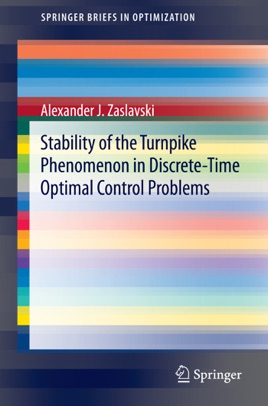• \$39.99

## Publisher Description

The structure of approximate solutions of autonomous discrete-time optimal control problems and individual turnpike results for optimal control problems without convexity (concavity) assumptions are examined in this book. In particular, the book focuses on the properties of approximate solutions which are independent of the length of the interval, for all sufficiently large intervals; these results apply to the so-called turnpike property of the optimal control problems. By encompassing the so-called turnpike property the approximate solutions of the problems are determined primarily by the objective function and are fundamentally independent of the choice of interval and endpoint conditions, except in regions close to the endpoints. This book also explores the turnpike phenomenon for two large classes of autonomous optimal control problems. It is illustrated that the turnpike phenomenon is stable for an optimal control problem if the corresponding infinite horizon optimal control problem possesses an asymptotic turnpike property. If an optimal control problem belonging to the first class possesses the turnpike property, then the turnpike is a singleton (unit set). The stability of the turnpike property under small perturbations of an objective function and of a constraint map is established. For the second class of problems where the turnpike phenomenon is not necessarily a singleton the stability of the turnpike property under small perturbations of an objective function is established. Containing solutions of difficult problems in optimal control and presenting new approaches, techniques and methods this book is of interest for mathematicians working in optimal control and the calculus of variations. It also can be useful in preparation courses for graduate students.